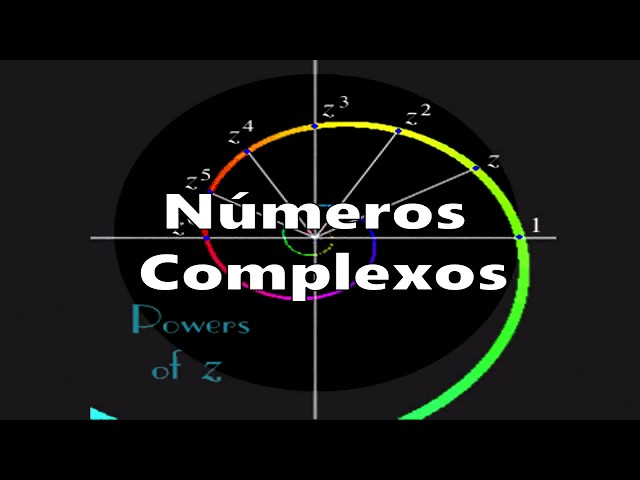# Introduction to Complex Analysis - Complex Numbers - Lesson 1 (Part 1)TLDR Complex numbers have a geometric interpretation in the xy plane and can be represented as vectors with a certain angle and size, allowing for operations such as addition and multiplication.

## Key insights

• 🧩
The concept of holomorphic functions will be explored in the course, including their properties and applications.
• 💡
The interpretation of complex number products was crucial for the development of complete analysis.

## Timestamped Summary

• 📚
00:00
In this video, the speaker introduces the complex analysis course, discussing the history and properties of complex numbers, as well as the concept of holomorphic functions and their applications.
• 📚
01:24
Complex numbers were invented to include the roots of negative numbers, and the quadratic equation has a solution, whether it is real or imaginary, with the line intersecting the parabola at two points when certain conditions are met.
• 📚
04:14
Complex numbers were discovered through the study of quadratic and cubic equations, with negative roots and complex numbers being used to find solutions.
• 📚
07:21
The speaker explains complex numbers and their arithmetic operations, highlighting addition and cube root calculation, and how a fireman devised a clever method to define these operations. The product of two complex numbers can be expressed as a sum and a product, with the result being either plus or minus one.
• 🧮
11:16
Complex numbers were initially mysterious to mathematicians, but in the late 18th century, mathematicians like Argand and Gauss gave them a geometric meaning, defining them as points on the xy plane.
• 📝
14:21
Complex numbers have a geometric interpretation in the xy plane, where the real part corresponds to the x-axis and the imaginary part corresponds to the y-axis, forming a vector with a certain angle and size.
• 📝
16:28
The absolute value of a complex number can be written as r times the cosine of theta plus i times the sine of theta, and when multiplying two complex numbers, the product is equal to the product of their absolute values times the cosine of the sum of their angles plus i times the sine of the sum of their angles.
• 📚
19:13
When multiplying complex numbers, you rotate the vector and expand it, with the product being a complex number with a new length and angle in relation to the x-axis.Report the articleThank you for the feedback